##### Differential Equations For DummiesOne of the cornerstones of calculus is the difference quotient. The difference quotient — along with limits — allows you to take the regular old slope formula that you used to compute the slope of lines in algebra class and use it for the calculus task of calculating the slope (or derivative) of a curve. Here’s how it works.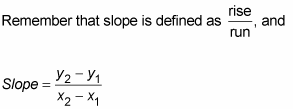In the following example, you want to find the slope at a point on the parabola.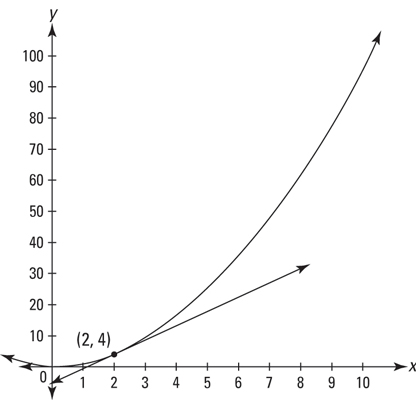To compute the slope, you need two points to plug into this formula. For a line, this is easy. You just pick any two points on the line and plug them in.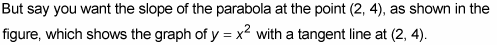You can see the line drawn tangent to the curve at (2, 4), and because the slope of the tangent line is the same as the slope of the parabola at (2, 4), all you need is the slope of the tangent line. But you don’t know the equation of the tangent line, so you can’t get the second point — in addition to (2, 4) — that you need for the slope formula.

Here’s how the inventors of calculus got around this roadblock.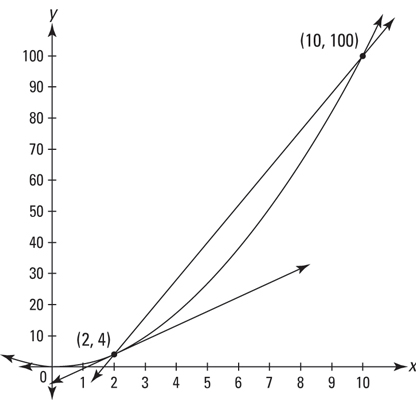The above figure is the graph of y = x2 with a tangent line and a secant line. It shows the tangent line again and a secant line intersecting the parabola at (2, 4) and at (10, 100).

A secant line is a line that intersects a curve at two points. This is a bit oversimplified, but it’ll do.

The slope of this secant line is given by the slope formula: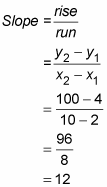You can see that this secant line is quite a bit steeper than the tangent line, and thus the slope of the secant, 12, is higher than the slope you’re looking for.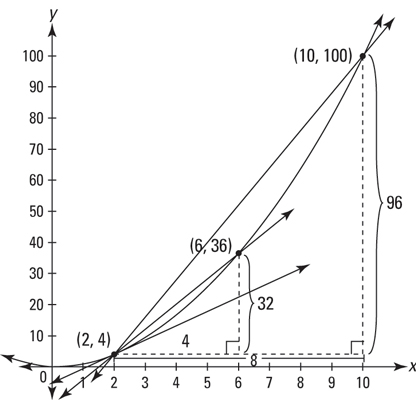Now add one more point at (6, 36) and draw another secant using that point and (2, 4) again. See the above figure.

Calculate the slope of this second secant: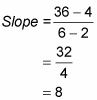You can see that the slope of this secant line is a better approximation of the slope of the tangent line than the slope of the first secant was.

Now, imagine what would happen if you grabbed the point at (6, 36) and slid it down the parabola toward (2, 4), dragging the secant line along with it. Can you see that as the point gets closer and closer to (2, 4), the secant line gets closer and closer to the tangent line, and that the slope of this secant thus gets closer and closer to the slope of the tangent?

So, you can get the slope of the tangent if you take the limit of the slope of this moving secant.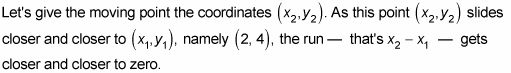So here’s the limit you need: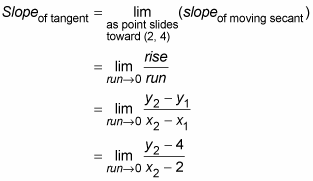Watch what happens to this limit when you plug in three more points on the parabola that are closer and closer to (2, 4):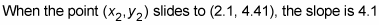When the point slides to (2.01, 4.0401), the slope is 4.01

When the point slides to (2.001, 4.004001), the slope is 4.001

Sure looks like the slope is headed toward 4.

As with all limit problems, the variable in this problem, the run, approaches but never actually gets to zero. If it got to zero — which would happen if you slid the point you grabbed along the parabola until it was actually on top of (2, 4) — you’d have a slope of 0/0, which is undefined. But, of course, that’s precisely the slope you want — the slope of the line when the point does land on top of (2, 4). Herein lies the beauty of the limit process.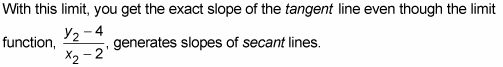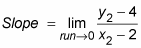And the slope of the tangent line is — you guessed it — the derivative.

The derivative of a function, f(x), at some number x = c, written as f’(c), is the slope of the tangent line to f drawn at c.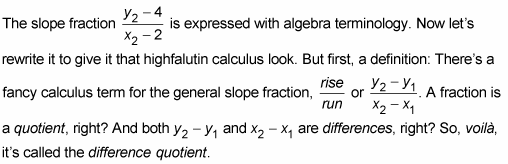Okay, here’s the most common way of writing the difference quotient (you may run across other, equivalent ways).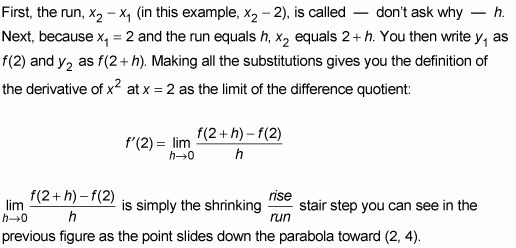Take a look at the following figure, which shows how a limit produces the slope of the tangent line at (2, 4).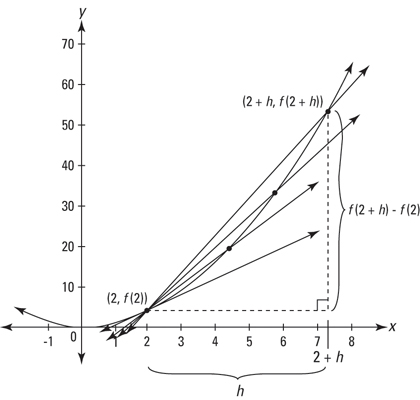Doing the math gives you, at last, the slope of the tangent line at (2, 4):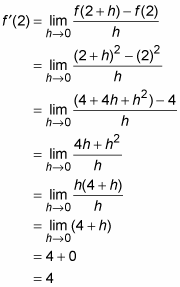So the slope is 4. (By the way, it’s a meaningless coincidence that the slope at (2, 4) happens to be the same as the y-coordinate of the point.)